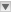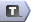Logic in AMPL

Relational Operators

Relational operators are used to compare two expressions. They are most commonly used in constraints, but not exclusively. The relational operators are:

Expression Meaning<> Not equal to
<= Less than or equal to
< Less than
>= Greater than or equal to
> Greater than
>= Equal to

Logical Expressions

Logical expressions are expressions that will evaluate to either true or false. Logical expressions are usually defined in terms of the relational operators:

Lower[r] <= sum {i in INGREDIENTS} Contributes[r, i] * Amount[i]

sum {s in SURFBOARDS} Recipe[m, s] * Production[s] <= Supply[m];
1. <e> in <SET> is true if <e> is a member of <SET>;
2. {\tt <e> not in <SET>} is false if <e> is a member of <SET>;
3. exists {<e> in <SET>} <expression> is true if some <e> in <SET> has <expression> being true;
4. forall {<e> in <SET>} <expression> is true if all <e> in <SET> have <expression> being true;
5. <SUBSET> within <SET> is true if all the elements in <SUBSET> are in <SET>;
6. <SUBSET> not within <SET> is true if some element in <SUBSET> is not in <SET>.

Logical expressions can be built up from other logical expressions, binary parameters and logical operators.

Binary Parameters

In AMPL we can create binary parameters by using the {\tt binary} keyword in the parameter declaration:

\begin{verbatim} param stillSearching binary; \end{verbatim}

Binary parameters are used in a similar way to boolean variables (in Matlab, C, etc) and logical variables (Fortran). If a binary parameter has the value 0 this is equivalent to false, and 1 is equivalent to true. Binary parameters can be used with conditional expressions to hold a true/false result from a logical expression:

\begin{verbatim} param isGreater binary;

let isGreater := if 4 > 5 then 1 else 0; # isGreater = 0 (false) let isGreater := if 6 > 5 then 1; # else 0 is the default, isGreater = 1 (true) \end{verbatim}

so the syntax is

\begin{verbatim} \end{verbatim} or as the condition in a conditional statement or conditional structure. They are very useful for building complex conditional statements or structures:

\begin{verbatim} Some example from depth first searching or column generation \end{verbatim}

and controlling loops.

Logical Operators

<\tt not} {\tt and} {\tt or}

Conditional Expressions

A conditional expression is very much like the IF function in Excel: param ifvalue := if then [else ];

Conditional Structures

A conditional structure is the same as the classical if-then-else statement in programming languages like MATLAB, Fortran, Visual Basic and C++: if then ; [else ;] Note here that even if the else keyword is present you need to end with ;. If you want to include more than one statement within the conditional structures you can use { and } to enclose your statements. if then { } [else { }]

-- TWikiAdminGroup - 18 Mar 2008

Edit | Attach | Watch | Print version |  | Backlinks | Raw View | Raw edit | More topic actions...
Topic revision: r3 - 2008-03-18 - TWikiAdminUserEdit AttachCopyright © 2008-2022 by the contributing authors. All material on this collaboration platform is the property of the contributing authors.
Ideas, requests, problems regarding TWiki? Send feedback